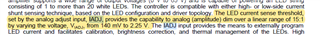If you have a related question, please click the "Ask a related question" button in the top right corner. The newly created question will be automatically linked to this question.

Part Number: TPS92691-Q1

Hi expert,

I checked from datasheet. Found that the description of IADJ pin as below. How to understand the linear range of 15:1. Is that mean IADJ only have 15 steps to control. The step is (2.25-0.24)/15=134mV. 92691 can not support linear adjust for IADJ? Am I misunderstand?Thanks!

• Hello,

The IADJ is really an analog input so there is continuous adjustment of brightness and not steps.  But the 15:1 range comes from (2.25 - 0.14) / 0.14 = 15.  This is the ratio of of dimming from brightest relative to dimmest.

Thanks Tuan

• Hi Tuan,

Sorry, I am confused. The IADJ is for brightness control, but 15:1 is the ratio of dimming from brightest relative to dimmest. What is the difference between brightness control and 15:1 ratio of dimming.

Thanks!

• Hello Chuqiang,

Another word if you set IADJ pin to 2.25V and measure the current on the LED and divide that with the LED current when IADJ is set to 140mV...The current ratio will be 15.  this is the dimming ratio.

Thanks Tuan